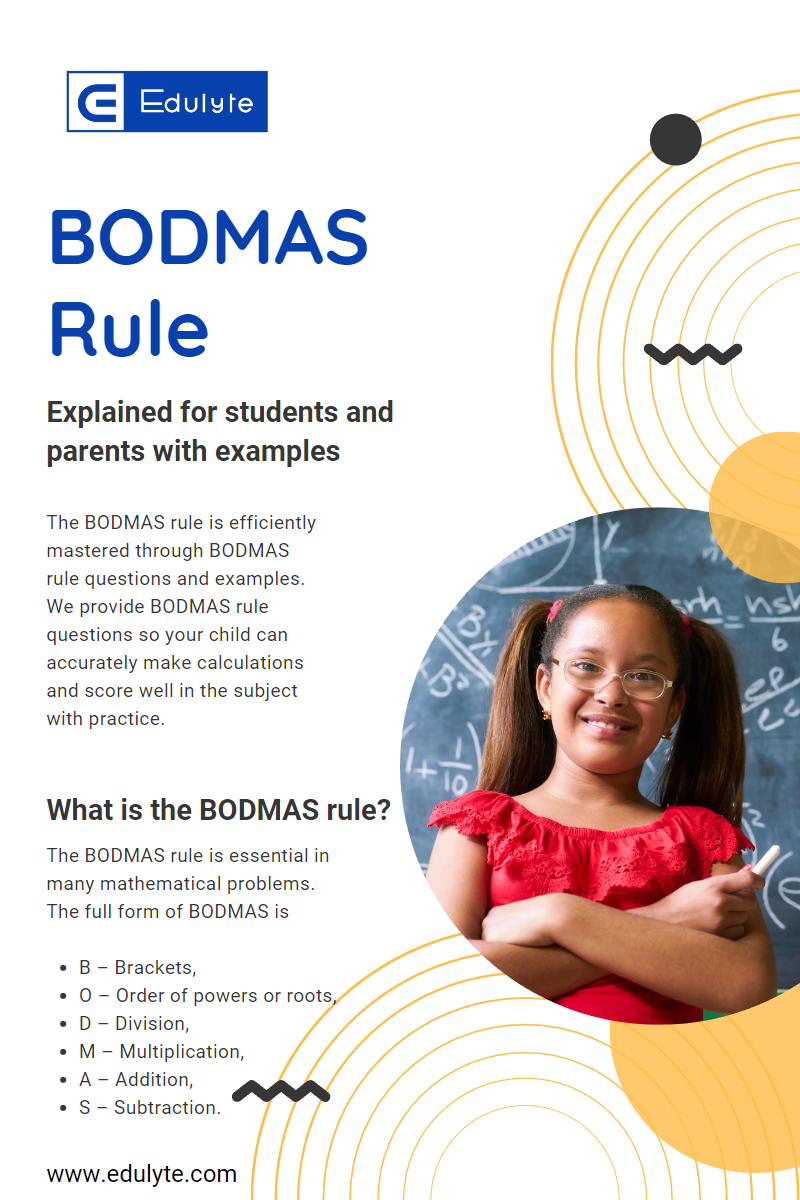Share
Explore### The BODMAS rule is efficiently mastered through BODMAS rule questions and examples. We provide BODMAS rule questions so your child can accurately make calculations and score well in the subject with practice.. Ok, what do we know about it? It’s an Acronym. It is used to solve arithmetic problems. And it has caused you many incorrect answers in the Maths class. The BODMAS rule guides the mathematical operations through the BODMAS sequence. Unfortunately, handling the BODMAS rule in Maths can get a little confusing. Edulyte’s (all Maths classes link once ready) Maths mentors have devised ways to save you from creating further disasters with the BODMAS formula and enable you to become a BODMAS wizard. Read on to find out!

# What is the BODMAS⁠ rule?

The BODMAS rule is essential in many mathematical problems. The full form of BODMAS is
B – Brackets,
O – Order of powers or roots,
D – Division,
M – Multiplication,
S – Subtraction.
S – Subtraction. The BODMAS theory solves problems that have multiple operations. So when you see addition, multiplication, subtraction, and division together, you are prepared to find the correct answer following the proper sequence of operations.

# When to use BODMAS⁠?

Maths is all about logical interpretations and systematic solving of problems. Hence it requires a specific set of procedures to be observed. It is easy to perform one function, like addition, with two or three numbers. But if a question involves more than one , it can be confusing to solve the problem without guidance.
You can utilise the BODMAS formula whenever there is more than one operation in a mathematical equation or expression. We have to follow the set sequence so that the results are correct. We cannot just calculate from left to right or in a haphazard manner. The BODMAS rule in Maths identifies the correct order or sequence to be followed while solving the maths problem.